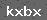# 600kw康明斯发电机每小时油耗

600kw康明斯柴油发电机组每小时耗油量=126公斤(kg) =157.5升 (L)

600kw发电机组耗油跟以下两个因素有关：

一、燃油消耗率，不同品牌的发电机组，其燃油消耗率不同，消耗油量就不同；

二、用电负载的大小，负载大了油门大耗油就大些，反之负载小了相对油耗也就要小些。

发电机的油耗量具体的计算方式如下：

一升柴油约等于0.84-0.86公斤（1L=0.8-0.85KG）左右。

发电机的制造商使用参数大多都会用G/KW.H，其意思是指发电机组一kw一小时耗多少克（G）油，再将单位换成升（L）从而就能知道你一小时耗油成本。

为方便大家了解发电机组的大致耗油量，计算发电机组的使用成本；给大家计算下大致的参考值（30kw—500kw）。

30kw发电机组油耗量=6.3公斤(kg) =7.8升(L)

45kw发电机组油耗量=9.45公斤(kg) =11.84升(L)

50kw发电机组油耗量=10.5公斤(kg) =13.1升 (L)

75kw发电机组油耗量=15.7公斤(kg) =19.7升 (L)

100kw发电机组油耗量=21公斤(kg) =26.25升 (L)

150kw发电机组油耗量=31.5公斤(kg) =39.4 升(L)

200kw发电机组油耗量=40公斤(kg) =50升(L)

250kw发电机组油耗量=52.5公斤(kg) =65.6升 (L)

300kw发电机组油耗量=63公斤(kg) =78.75升 (L)

350kw发电机组油耗量=73.5公斤(kg) =91.8升(L)

400kw发电机组油耗量=84.00公斤(kg) =105.00 升(L)

450kw发电机组油耗量=94.50公斤(kg) =118.00升 (L)

500kw发电机组油耗量=105.00公斤(kg) =131.20升 (L)

以上只是估算值，仅供参考。TAGS:

### 发表评论

 留言与评论（共有 0 条评论）

 验证码：Q Q：1319258423